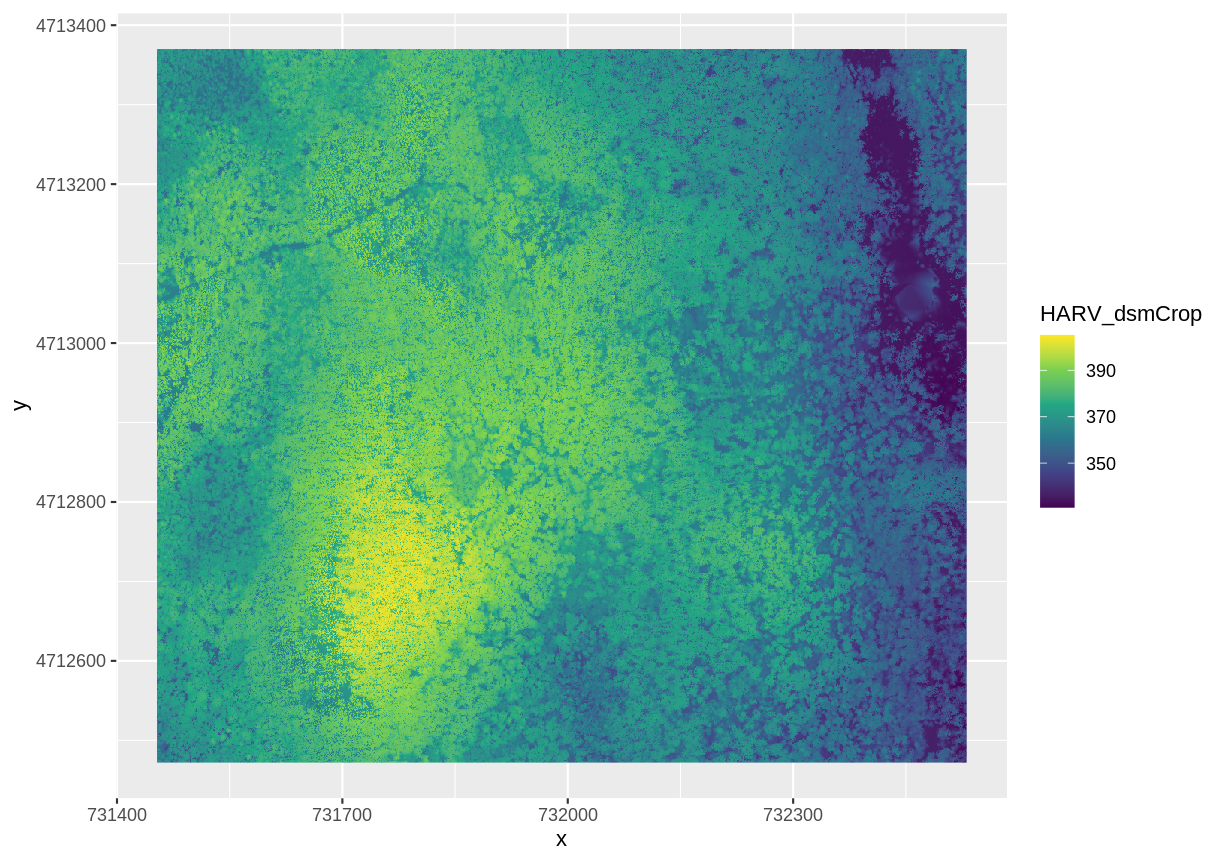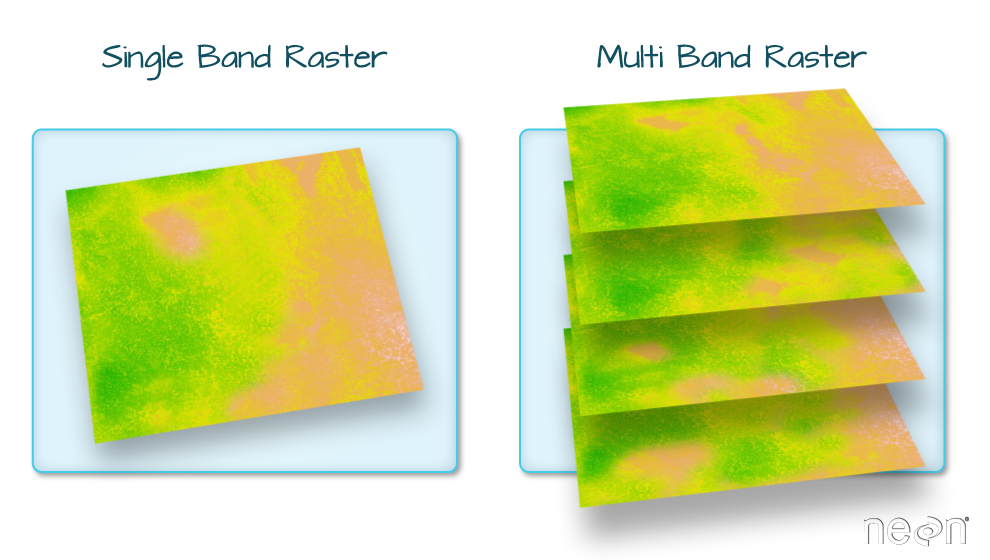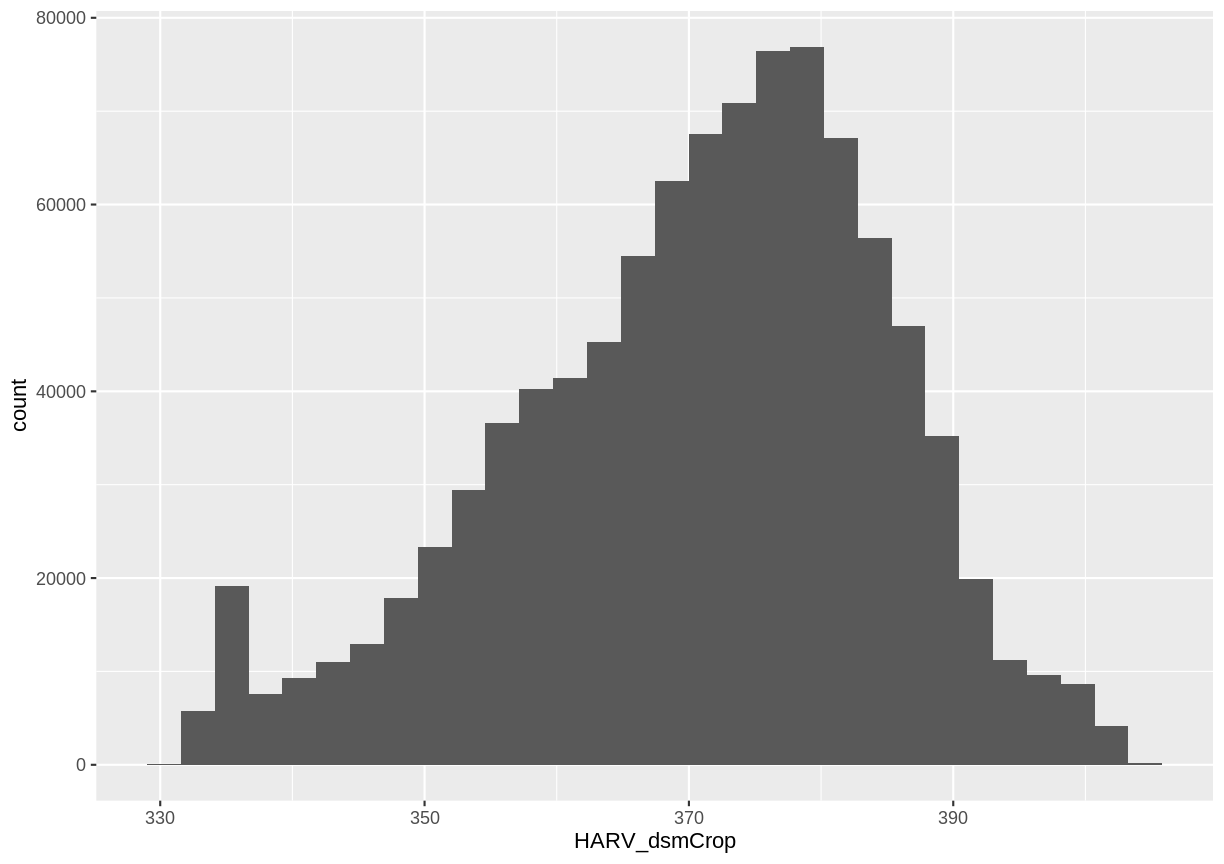This lesson is being piloted (Beta version)

# Intro to Raster Data in R

## Overview

Teaching: 40 min
Exercises: 20 min
Questions
• What is a raster dataset?

• How do I work with and plot raster data in R?

• How can I handle missing or bad data values for a raster?

Objectives
• Describe the fundamental attributes of a raster dataset.

• Explore raster attributes and metadata using R.

• Import rasters into R using the `raster` package.

• Plot a raster file in R using the `ggplot2` package.

• Describe the difference between single- and multi-band rasters.

In this episode, we will introduce the fundamental principles, packages and metadata/raster attributes that are needed to work with raster data in R. We will discuss some of the core metadata elements that we need to understand to work with rasters in R, including CRS and resolution. We will also explore missing and bad data values as stored in a raster and how R handles these elements.

We will continue to work with the `dplyr` and `ggplot2` packages that were introduced in the Introduction to R for Geospatial Data lesson. We will use two additional packages in this episode to work with raster data - the `raster` and `rgdal` packages. Make sure that you have these packages loaded.

``````library(raster)
library(rgdal)
``````

##The Data

In this lesson, we will be working with two field sites: the Harvard Forest (`HARV`) and San Joaquin Experimental Range (`SJER`).

In this lesson, the raster we will use is: `HARV_dsmCrop.tif`.

For the challenges in this lesson, we will use both and `HARV_dsmCrop.tif` and `HARV_DSMhill.tif`.

## View Raster File Attributes

We will be working with a series of GeoTIFF files in this lesson. The GeoTIFF format contains a set of embedded tags with metadata about the raster data. We can use the function `GDALinfo()` to get information about our raster data before we read that data into R. It is ideal to do this before importing your data.

``````GDALinfo("data/raster/HARV_dsmCrop.tif")
``````
``````rows        898
columns     1078
bands       1
lower left origin.x        731453
lower left origin.y        4712472
res.x       1
res.y       1
ysign       -1
oblique.x   0
oblique.y   0
driver      GTiff
projection  +proj=utm +zone=18 +datum=WGS84 +units=m +no_defs
file        data/raster/HARV_dsmCrop.tif
apparent band summary:
GDType hasNoDataValue NoDataValue blockSize1 blockSize2
1 Float32           TRUE    -3.4e+38          1       1078
apparent band statistics:
Bmin   Bmax    Bmean      Bsd
1 330.9 405.15 370.6424 14.27794
Metadata:
AREA_OR_POINT=Area
``````

If you wish to store this information in R, you can do the following:

``````HARV_dsmCrop_info <- capture.output(
GDALinfo("data/raster/HARV_dsmCrop.tif")
)
HARV_dsmCrop_info
``````
``````  "rows        898 "
 "columns     1078 "
 "bands       1 "
 "lower left origin.x        731453 "
 "lower left origin.y        4712472 "
 "res.x       1 "
 "res.y       1 "
 "ysign       -1 "
 "oblique.x   0 "
 "oblique.y   0 "
 "driver      GTiff "
 "projection  +proj=utm +zone=18 +datum=WGS84 +units=m +no_defs "
 "file        data/raster/HARV_dsmCrop.tif "
 "apparent band summary:"
 "   GDType hasNoDataValue NoDataValue blockSize1 blockSize2"
 "1 Float32           TRUE    -3.4e+38          1       1078"
 "apparent band statistics:"
 "   Bmin   Bmax    Bmean      Bsd"
 "1 330.9 405.15 370.6424 14.27794"
 "Metadata:"
 "AREA_OR_POINT=Area "
``````

Each line of text that was printed to the console is now stored as an element of the character vector `HARV_dsmCrop_info`. We will be exploring this data throughout this episode. By the end of this episode, you will be able to explain and understand the output above.

## Open a Raster in R

Now that we’ve previewed the metadata for our GeoTIFF, let’s import this raster dataset into R and explore its metadata more closely. We can use the `raster()` function to open a raster in R.

First we will load our raster file into R and view the data structure.

``````DSM_HARV <- raster("data/raster/HARV_dsmCrop.tif")

DSM_HARV
``````
``````class      : RasterLayer
dimensions : 898, 1078, 968044  (nrow, ncol, ncell)
resolution : 1, 1  (x, y)
extent     : 731453, 732531, 4712472, 4713370  (xmin, xmax, ymin, ymax)
crs        : +proj=utm +zone=18 +datum=WGS84 +units=m +no_defs +ellps=WGS84 +towgs84=0,0,0
source     : /home/travis/build/UW-Madison-DataScience/r-raster-vector-geospatial/_episodes_rmd/data/raster/HARV_dsmCrop.tif
names      : HARV_dsmCrop
values     : 330.9, 405.15  (min, max)
``````

The information above includes a report of min and max values, but no other data range statistics. Similar to other R data structures like vectors and data frame columns, descriptive statistics for raster data can be retrieved like

``````summary(DSM_HARV)
``````
``````Warning in .local(object, ...): summary is an estimate based on a sample of 1e+05 cells (10.33% of all cells)
``````
``````        HARV_dsmCrop
Min.          331.05
1st Qu.       361.58
Median        372.57
3rd Qu.       380.80
Max.          404.77
NA's            0.00
``````

but note the warning - unless you force R to calculate these statistics using every cell in the raster, it will take a random sample of 100,000 cells and calculate from that instead. To force calculation on more, or even all values, you can use the parameter `maxsamp`:

``````summary(DSM_HARV, maxsamp = ncell(DSM_HARV))
``````
``````        HARV_dsmCrop
Min.          330.90
1st Qu.       361.52
Median        372.52
3rd Qu.       380.84
Max.          405.15
NA's            0.00
``````

You may not see major differences in summary stats as `maxsamp` increases, except with very large rasters.

To visualise this data in R using `ggplot2`, we need to convert it to a dataframe. The `raster` package has an built-in function for conversion to a plotable dataframe.

``````DSM_HARV_df <- as.data.frame(DSM_HARV, xy = TRUE)
``````

Now when we view the structure of our data, we will see a standard dataframe format. We can apply `str` to view the structure of the new dataframe, `DSM_HARV_df`

``````str(DSM_HARV_df)
``````
``````'data.frame':	968044 obs. of  3 variables:
\$ x           : num  731454 731454 731456 731456 731458 ...
\$ y           : num  4713370 4713370 4713370 4713370 4713370 ...
\$ HARV_dsmCrop: num  370 371 371 362 370 ...
``````

We can use `ggplot()` to plot this data. We will set the color scale to `scale_fill_viridis_c` which is a color-blindness friendly color scale. We will also use the `coord_quickmap()` function to use an approximate Mercator projection for our plots. This approximation is suitable for small areas that are not too close to the poles. Other coordinate systems are available in ggplot2 if needed, you can learn about them at their help page `?coord_map`.

``````ggplot() +
geom_raster(data = DSM_HARV_df , aes(x = x, y = y, fill = HARV_dsmCrop)) +
scale_fill_viridis_c() +
coord_quickmap()
``````This map shows the elevation of our study site in Harvard Forest. From the legend, we can see that the maximum elevation is ~400, but we can’t tell whether this is 400 feet or 400 meters because the legend doesn’t show us the units. We can look at the metadata of our object to see what the units are. Much of the metadata that we’re interested in is part of the CRS..

Now we will see how features of the CRS appear in our data file and what meanings they have.

### View Raster Coordinate Reference System (CRS) in R

We can view the CRS string associated with our R object using the`crs()` function.

``````crs(DSM_HARV)
``````
``````CRS arguments:
+proj=utm +zone=18 +datum=WGS84 +units=m +no_defs +ellps=WGS84
+towgs84=0,0,0
``````

## Challenge

What units are our data in?

## Answers

`+units=m` tells us that our data is in meters.

## Understanding CRS in Proj4 Format

The CRS for our data is given to us by R in `proj4` format. Let’s break down the pieces of `proj4` string. The string contains all of the individual CRS elements that R or another GIS might need. Each element is specified with a `+` sign, similar to how a `.csv` file is delimited or broken up by a `,`. After each `+` we see the CRS element being defined. For example projection (`proj=`) and datum (`datum=`).

### UTM Proj4 String

Our projection string for `DSM_HARV` specifies the UTM projection as follows:

`+proj=utm +zone=18 +datum=WGS84 +units=m +no_defs +ellps=WGS84 +towgs84=0,0,0`

• proj=utm: the projection is UTM, UTM has several zones.
• zone=18: the zone is 18
• datum=WGS84: the datum is WGS84 (the datum refers to the 0,0 reference for the coordinate system used in the projection)
• units=m: the units for the coordinates are in meters
• ellps=WGS84: the ellipsoid (how the earth’s roundness is calculated) for the data is WGS84

Note that the zone is unique to the UTM projection. Not all CRS’s will have a zone. Image source: Chrismurf at English Wikipedia, via Wikimedia Commons (CC-BY).## Calculate Raster Min and Max Values

It is useful to know the minimum or maximum values of a raster dataset. In this case, given we are working with elevation data, these values represent the min/max elevation range at our site.

Raster statistics are often calculated and embedded in a GeoTIFF for us. We can view these values:

``````minValue(DSM_HARV)
``````
`````` 330.9
``````
``````maxValue(DSM_HARV)
``````
`````` 405.15
``````

Since the minimum and maximum values haven’t already been calculated, we can calculate them using the `setMinMax()` function.

``````DSM_HARV <- setMinMax(DSM_HARV)
minValue(DSM_HARV)
``````
`````` 330.9
``````
``````maxValue(DSM_HARV)
``````
`````` 405.15
``````

## Raster Bands

The Digital Surface Model object (`DSM_HARV`) that we’ve been working with is a single band raster. This means that there is only one dataset stored in the raster: surface elevation in meters for one time period.A raster dataset can contain one or more bands. We can use the `raster()` function to import one single band from a single or multi-band raster. We can view the number of bands in a raster using the `nlayers()` function.

``````nlayers(DSM_HARV)
``````
`````` 1
``````

However, raster data can also be multi-band, meaning that one raster file contains data for more than one variable or time period for each cell. By default the `raster()` function only imports the first band in a raster regardless of whether it has one or more bands.

##Missing Data

The value that is conventionally used to take note of missing data (the `NoDataValue` value) varies by the raster data type. For floating-point rasters, the figure `-3.4e+38` is a common default, and for integers, `-9999` is common. Some disciplines have specific conventions that vary from these common values.

In some cases, other `NA` values may be more appropriate. An `NA` value should be a) outside the range of valid values, and b) a value that fits the data type in use. For instance, if your data ranges continuously from -20 to 100, 0 is not an acceptable `NA` value! Or, for categories that number 1-15, 0 might be fine for `NA`, but using -.000003 will force you to save the GeoTIFF on disk as a floating point raster, resulting in a bigger file.

If we are lucky, our GeoTIFF file has a tag that tells us what is the `NoDataValue`. If we are less lucky, we can find that information in the raster’s metadata. If a `NoDataValue` was stored in the GeoTIFF tag, when R opens up the raster, it will assign each instance of the value to `NA`. Values of `NA` will be ignored by R as demonstrated above.

## Challenge

Use the output from the `GDALinfo()` function to find out what `NoDataValue` is used for our `DSM_HARV` dataset.

## Answers

``````GDALinfo("data/raster/HARV_dsmCrop.tif")
``````
``````rows        898
columns     1078
bands       1
lower left origin.x        731453
lower left origin.y        4712472
res.x       1
res.y       1
ysign       -1
oblique.x   0
oblique.y   0
driver      GTiff
projection  +proj=utm +zone=18 +datum=WGS84 +units=m +no_defs
file        data/raster/HARV_dsmCrop.tif
apparent band summary:
GDType hasNoDataValue NoDataValue blockSize1 blockSize2
1 Float32           TRUE    -3.4e+38          1       1078
apparent band statistics:
Bmin   Bmax    Bmean      Bsd
1 330.9 405.15 370.6424 14.27794
Metadata:
AREA_OR_POINT=Area
``````

`NoDataValue` are encoded as -9999.

## Create A Histogram of Raster Values

We can explore the distribution of values contained within our raster using the `geom_histogram()` function which produces a histogram. Histograms are often useful in identifying outliers and bad data values in our raster data.

``````ggplot() +
geom_histogram(data = DSM_HARV_df, aes(HARV_dsmCrop))
``````
```````stat_bin()` using `bins = 30`. Pick better value with `binwidth`.
``````Notice that a warning message is thrown when R creates the histogram.

`stat_bin()` using `bins = 30`. We could pick a better bin value with the argument `binwidth`.

## Challenge: Explore Raster Metadata

Use `GDALinfo()` to determine the following about the `data/raster/HARV_DSMhill.tif` file:

1. Does this file have the same CRS as `DSM_HARV`?
2. What is the `NoDataValue`?
3. What is resolution of the raster data?
4. How large would a 5x5 pixel area be on the Earth’s surface?
5. Is the file a multi- or single-band raster?

Notice: this file is a hillshade. We will learn about hillshades in the Working with Multi-band Rasters in R episode.

## Answers

``````GDALinfo("data/raster/HARV_DSMhill.tif")
``````
``````rows        898
columns     1078
bands       1
lower left origin.x        731453
lower left origin.y        4712472
res.x       1
res.y       1
ysign       -1
oblique.x   0
oblique.y   0
driver      GTiff
projection  +proj=utm +zone=18 +datum=WGS84 +units=m +no_defs
file        data/raster/HARV_DSMhill.tif
apparent band summary:
GDType hasNoDataValue NoDataValue blockSize1 blockSize2
1 Float32           TRUE    -3.4e+38          1       1078
apparent band statistics:
Bmin      Bmax     Bmean      Bsd
1 -0.7131745 0.9999997 0.2932656 0.487991
Metadata:
AREA_OR_POINT=Area
``````
1. If this file has the same CRS as DSM_HARV? Yes: UTM Zone 18, WGS84, meters.
2. What format `NoDataValues` take? -3.4e+38
3. The resolution of the raster data? 1x1
4. How large a 5x5 pixel area would be? 5mx5m How? We are given resolution of 1x1 and units in meters, therefore resolution of 5x5 means 5x5m.
5. Is the file a multi- or single-band raster? Single.

## Key Points

• The GeoTIFF file format includes metadata about the raster data.

• To plot raster data with the `ggplot2` package, we need to convert it to a dataframe.

• R stores CRS information in the Proj4 format.

• Be careful when dealing with missing or bad data values.